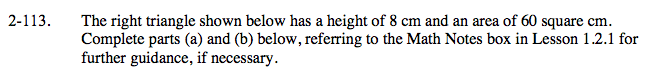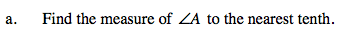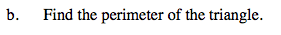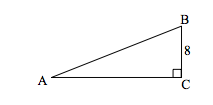### Home > CAAC > Chapter 14 > Lesson 14.2.1.8 > Problem2-113

2-113.Start by finding the length of the base of the triangle.

Use the tangent ratio to find the measure of angle A.

m∠A ≈ 28.07Use the Pythagorean Theorem to find the length of the hypotenuse.

Perimeter = 40 cm